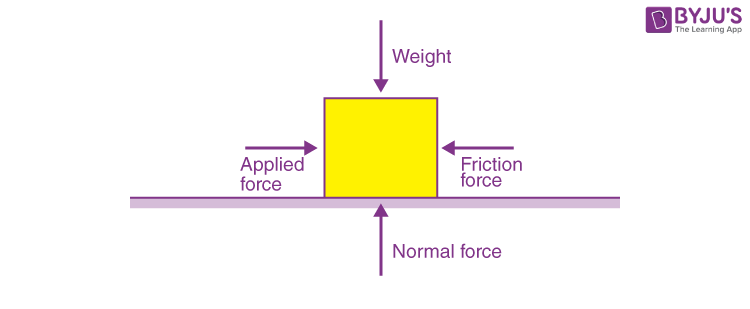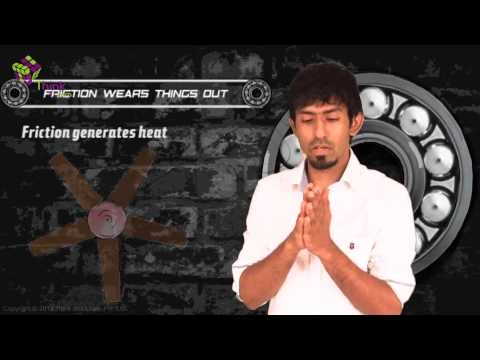# Contact Force

If you leave your ball on your table and go to sleep, will it be where you left it when you wake up? Of course, it will. A ball cannot move unless you make it move. In the situation when the force is exerted on objects which are in physical contact with each other, the force applied is called contact force. This is the situation in many places, a book will not flip its pages unless you flip it yourself. A football will not fly into the goal unless you kick it. This brings us to the concept of force. Sir Isaac Newton gave us the concept of force. He said that an object would be in its position either in a static state or moving state until and unless an external force is applied to it. Force is a stimulus provided to an object in order to make it do something. Here the force can be both against the motion and for it. The amount of force required is related to the mass of the object, the greater the mass, the greater the force required to move it. Force can be classified into two groups.

• Contact Force
• Non-Contact Force

## Contact Force

Contact force is a force that is applied by objects in contact with each other. The contact force acts on a point of direct contact between the two objects. This force can either be continuous as a continuous force or can be momentary in the form of an impulse. Contact force is governed by Newton’s Laws. Contact force is responsible for most of the interactions we experience in daily life. Pushing a car up the hill, writing your name with a pen, or picking up a textbook from the table are all examples of contact force, and they are also examples of continuous force. On the other hand, hammering a nail, kicking a ball, etc are examples of impulse or momentary force.

### Types of contact force:

1. Frictional Force: Friction is a force exerted by a surface against the motion of a body across its surface. Friction is the reason why your car comes to a standstill if you don’t press the accelerator. The frictional force is opposing the rotation of the wheels of your car. Therefore, if you leave the accelerator, the friction slowly removes all the force from the car and when it has removed the last bit of force, the car comes to a standstill. Another example is, if a book slides across the surface of a desk, then the desk exerts a friction force in the opposite direction of its motion causing it to stop sliding after a finite distance. Friction is a type of contact force only.
2. Applied Force: Force which is applied to an object by another object. A person pushing a barrel is an example of applied force. When the person pushes the barrel then there is an applied force acting upon the barrel. The applied force is the force exerted on the barrel by the person and it is responsible for the motion of the barrel. The applied force can be both a contact force or a non-contact force.
3. Normal Force: The normal force is also called support force. The normal force is the support force exerted upon an object that is in contact with another fixed or stable object. For example, if a book is resting upon a surface, then the surface is exerting an upward force upon the book in order to support the weight of the book. The solid ground provides the normal force for all of us live. The normal force is proportional to the weight of the body. Larger the body, the larger the normal force. If a very high weight is kept on a table and it cannot provide a normal force that large, then it breaks.In this article, you glanced through the concept of force and took an in-depth look at contact force and its types, namely, frictional force, applied force and Normal force. Other types of contact forces outside the scope of syllabus include Air Resistance Force, Tension Force and Spring Force. Watch interactive videos on force and other concepts of physics, download BYJU’S The Learning App.

Meanwhile, watch this video to know more about friction. We have more such videos on our APP to clear your concepts. Just download the app and enjoy the process of learning.Test Your Knowledge On Force Contact Force Non Contact Force!